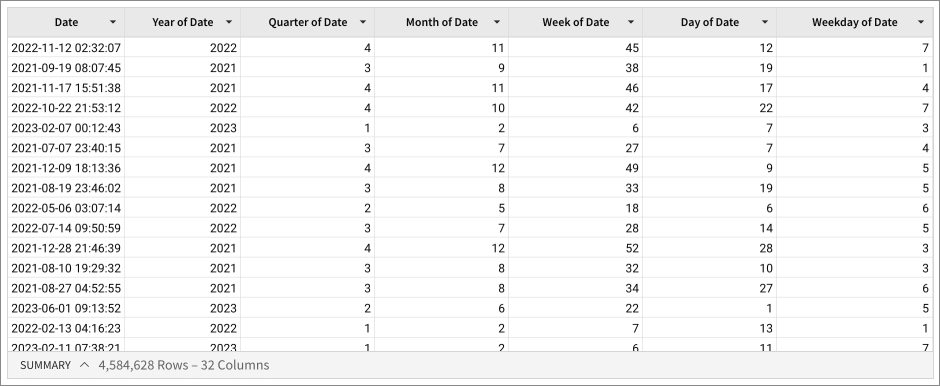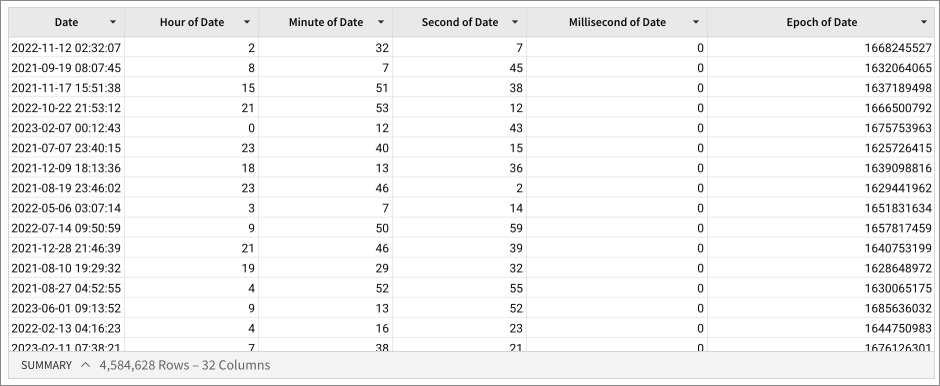# DatePart

The DatePart function extracts the specified date part from a date value.

See Sigma's Common Date Functions and Use Cases lab for more information about date functions, including use cases and exercises.

Sigma returns the result(s) in your organization's time zone. This may cause days, months, or years to appear offset, if the specified time zone is ahead of the organization's time zone. To view the output presented in the specified time zone, apply the ConvertTimezone function.

DatePart is one of Sigma's Date functions.

## Syntax

`DatePart(precision, date, [timezone])`

The function has the following arguments:

precision
Required
The date part extracted. Can be one of “year”, "quarter", “month”, “week”, “day”, "weekday", “hour”, “minute”, “second”, “millisecond”, or "epoch".
date
Required
Date or column of dates where Sigma etracts the date part
Note that the value must be a date. If the column is not in the appropriate format, use the Date function on the argument.
timezone
Optional
Name of IANA time zone for the date part. For example, ”America/Los_Angeles”.
If omitted, Sigma treats input dates as UTC.

## Examples

`DatePart("year", [Invoice Date])`

Extracts the year from each value in the column of invoice dates.

`DatePart("week", Date("2007-01-10 10:00:00"))`

Returns 2, the week number of this date; weeks in Sigma start on Sunday, by default.

```DatePart("year", Date([Date]))DatePart("quarter", Date([Date]))DatePart("month", Date([Date]))
DatePart("week", Date([Date]))DatePart("day", Date([Date]))DatePart("weekday", Date([Date]))```

The DatePart function returns the following values for the Date column:```DatePart("year", Date([Date]))
DatePart("hour", Date([Date]))DatePart("minute", Date([Date]))DatePart("second", Date([Date]))DatePart("millisecond", Date([Date]))DatePart("epoch", Date([Date]))```

The DatePart function returns the following values for the Date column: